# Equality of Opposite angles when Two sides of a Triangle are equal

If two sides of a triangle are equal, then the angles opposite to the same two sides are also equal.

## Introduction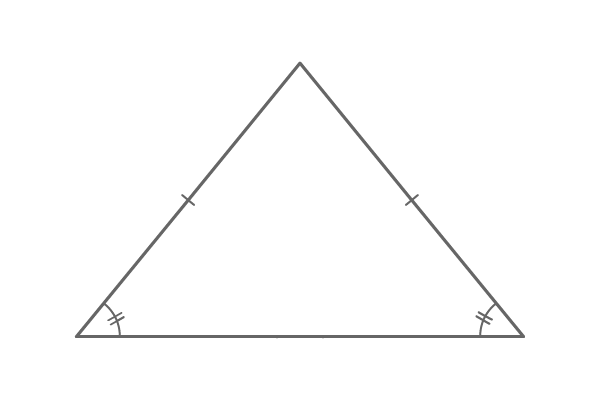The lengths of two sides in a triangle can be equal. It is possible only in the case of both equilateral triangle and isosceles triangle.

Due to the equality property of two sides in the triangle, the angles that are opposite to them are also equal geometrically. This theorem can also be proved in geometry on the basis of symmetry property.

Therefore, it is cleared that the angles opposite to the two sides of equal length are equal in a triangle.

### Proof

For proving this theorem, we have to construct either an equilateral or isosceles triangle.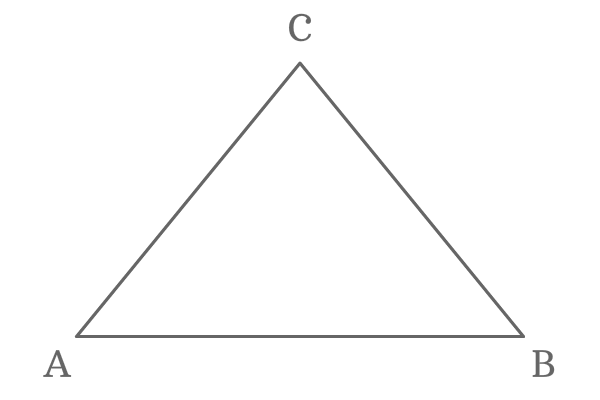$\Delta ABC$ is a triangle and its two sides are of equal length. Hence, the triangle $ABC$ is called an isosceles triangle. In this isosceles triangle, the lengths of the sides $\overline{AC}$ and $\overline{BC}$ are equal.

$AC = BC$

Draw a perpendicular line to the side $\overline{AB}$ from point $C$. It divides the side $\overline{AB}$ at its middle point exactly and the point of intersection is $D$.

Therefore, $AD = BD$.

Similarly, the line $\overline{DC}$ divides the $\Delta ABC$ as two right angled triangles $\Delta DAC$ and $\Delta DBC$. Now, the perpendicular line $\overline{DC}$ is a common side to both right angled triangles.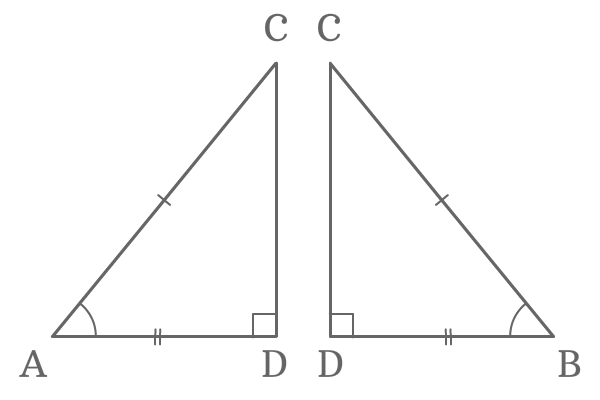Now, compare the lengths of three sides of both triangles.

1. $AC = BC$
2. $AD = BD$
3. $DC = DC$

The comparison of lengths of sides of the both triangles revealed that the two triangles are same but represented differently.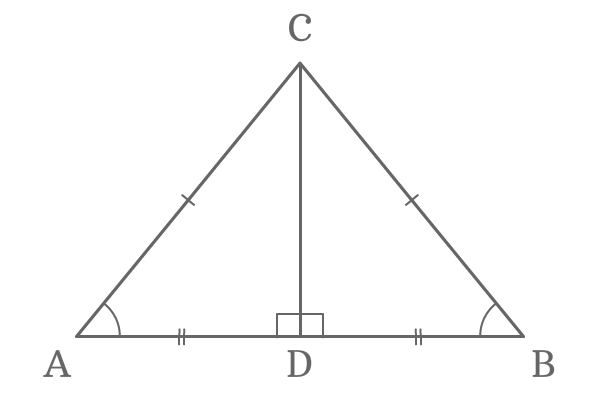Therefore, the $\Delta DAC$ and $\Delta DBC$ are known as congruent triangles and they also represent Right angle Hypotenuse side (RHS) criterion.

$\Delta DAC \cong \Delta DBC$

In this case, $\angle DAC$ is the opposite angle to the side $\overline{BC}$ and $\angle DBC$ is also opposite angle to the side $\overline{AC}$. The two angles are equals due to the congruency.

$\angle DAC = \angle DBC$

Therefore, if two sides of a triangle are equal, then the angles opposite to them are also equal.

#### Example

This property of the triangle can be proved geometrically by constructing a triangle but the lengths of any two sides of the triangle should be equal.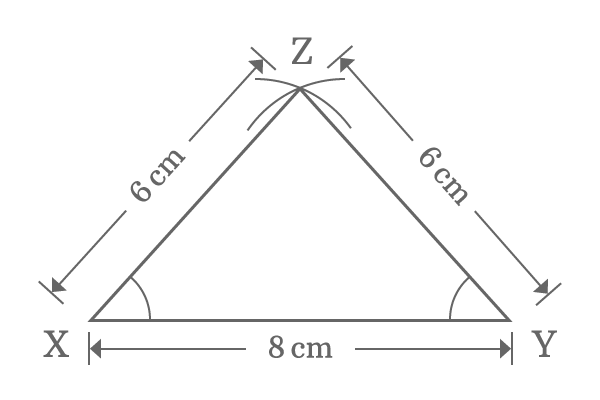Take ruler and draw a straight line ($\overline{XY}$) of length $8 \, cm$ horizontally.

Use compass and set distance between needle point and pencil point to $6 \, cm$. Draw an arc from point $X$ and also draw another arc from point $Y$ but the arcs should be intersected. The intersecting point of both arcs is $Z$.

Join points $X$ and $Z$ and also join points $Y$ and $Z$ by a straight line using ruler. Thus, the $\Delta XYZ$ is constructed geometrically.

$\angle XYZ$ and $\angle YXZ$ are opposite angles of the equal length sides $\overline{XZ}$ and $\overline{YZ}$ respectively. Now, measure the angles $XYZ$ and $YXZ$ by using protractor.

You practically observe that the $\angle XYZ$ and $\angle YXZ$ are equal and it is $48^\circ$ geometrically. Therefore, the angles opposite to the two equal length sides are equal geometrically.

Latest Math Topics
Jun 26, 2023
Jun 23, 2023

###### Math Questions

The math problems with solutions to learn how to solve a problem.

Learn solutions

Practice now

###### Math Videos

The math videos tutorials with visual graphics to learn every concept.

Watch now

###### Subscribe us

Get the latest math updates from the Math Doubts by subscribing us.